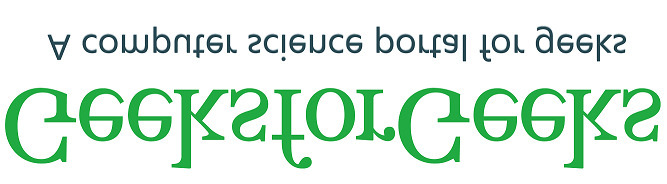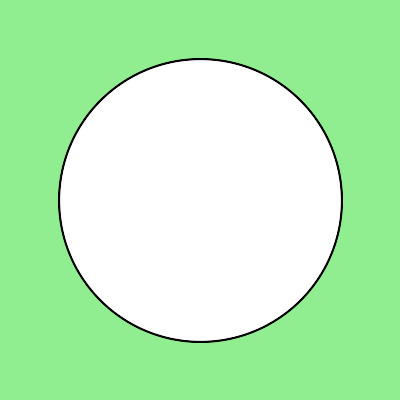Related Articles
Python Wand – An Overview
• Last Updated : 17 May, 2020

The Wand is an Imagick library for python. It supports the functionalities of Imagick API in Python 2.6, 2.7, 3.3+, and PyPy.This library not only helps in processing the images but also provides valuable functionalities for Machine Learning codes using NumPy.

Installation:
By Pip:

``\$ pip install Wand``

As the wand is an Imagick API, so we require Imagick dependencies.

Imagick’s Installation:

• For Ubuntu/Debian:
``\$ sudo apt-get install libmagickwand-dev``
• For Mac (By Brew Installer)

``\$ brew install imagemagick``

Installation of MacPorts

``\$ sudo port install imagemagick``

Note: If Python is not installed using MacPorts, we need to export MAGICK_HOME.

``\$ export MAGICK_HOME=/opt/local``
Example 1: Reading an Image:
Input Image: geeksforgeeks.png`# Import library from the wand``from` `wand.image ``import` `Image`` ` `# Import the image``with Image(filename ``=``'geeksforgeeks.png'``) as pic:`` ` `    ``# Read the image to fetch actual dimensions``    ``print``(``'Width of the image:'``, pic.width)``    ``print``(``'Height of the image:'``, pic.height)`

Output:

```('Width of the image:', 667L)
('Height of the image:', 184L)
```

Example 2: Blur an Image:

 `# Import library from the wand ``from` `wand.image ``import` `Image`` ` `# Import the image``with Image(filename ``=``"geeksforgeeks.png"``) as pic:`` ` `    ``# Invoke blur function with radius 0 and sigma 3``    ``pic.blur(radius ``=` `0``, sigma ``=` `3``)`` ` `    ``# save the processed iamge``    ``pic.save(filename ``=``"blur1.png"``)`

Output:Example 3: Transfor the image

 `# Import library from the wand``from` `wand.image ``import` `Image`` ` `# Import the image``with Image(filename ``=``'geeksforgeeks.png'``) as image:``    ``# Clone the image in order to process``    ``with image.clone() as flip:`` ` `        ``# Invoke flip function``        ``flip.flip()`` ` `        ``# Save the image``        ``flip.save(filename ``=``'flip-geeksforgeeks.jpg'``)`

Output:Example 4: Drawing:

 `# Import libraries from the wand  ``from` `wand.image ``import` `Image``from` `wand.drawing ``import` `Drawing``from` `wand.color ``import` `Color`` ` `with Drawing() as draw:``    ``# Set Stroke color the circle to black``    ``draw.stroke_color ``=` `Color(``'black'``)`` ` `    ``# Set Width of the circlw to 2 ``    ``draw.stroke_width ``=` `2`` ` `    ``# Set the fill color to 'White (# FFFFFF)'``    ``draw.fill_color ``=` `Color(``'white'``)`` ` `    ``# Invoke Circle function with center ``    ``# at 200, 200 and radius 100``    ``draw.circle((``200``, ``200``), ``# Center point``                ``(``100``, ``100``)) ``# Perimeter point``    ``with Image(width ``=` `400``, height ``=` `400``, ``              ``background ``=` `Color(``'lightgreen'``)) as pic:``        ``draw(pic)``        ``pic.save(filename ``=``'circle1.jpg'``)`

Output:References:

Attention geek! Strengthen your foundations with the Python Programming Foundation Course and learn the basics.

To begin with, your interview preparations Enhance your Data Structures concepts with the Python DS Course.

My Personal Notes arrow_drop_up